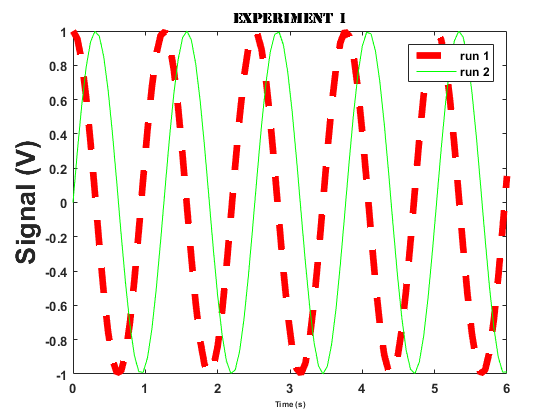# Homework #2

All problems must be in a single *.m file. You will need an additional file for a function. Make a separate block in the code for each problem.

## Problem 1

Create a function using a '*.m' file (see naming conventions) that divides the first input by the second and returns the result as the output. Demonstrate its use where the first input is 10 and the second input is 5.

## Problem 2

Create an anonymous function that performs identically to that in Problem 1

## Problem 3

Generate code that reproduces the following figure as closely as possible (close counts). Note the various font sizes and line types.## Problem 4

Recreate the Airy Disk plot shown here: https://en.wikipedia.org/wiki/Airy_disk#/media/File:Airy-3d.svg

`Hint: https://en.wikipedia.org/wiki/Airy_disk`
`Hint: (J1(0)/0)^2 = 0.25`
`Hint 'doc mesh'`
`Hint 'lookfor bessel'`